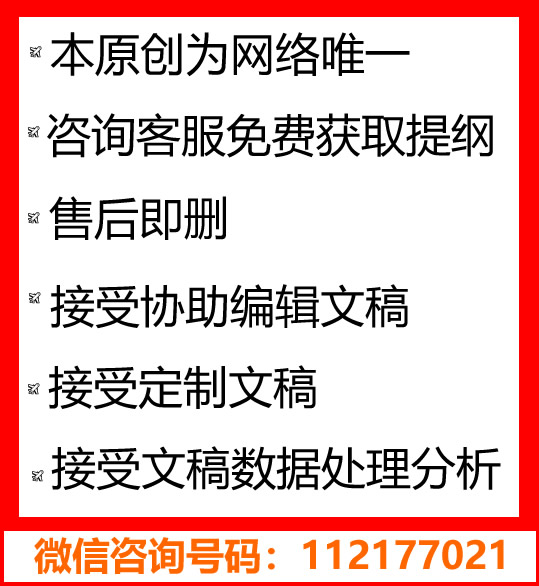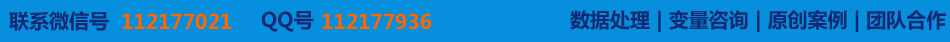# 不等式证明的若干方法

### 〖类型:案例|7000字， 类别:原创|系数:3.36， 附件：开题报告|文献综述〗

【摘要】

ABSTRACT

In elementary mathematics learning and learning in higher mathematics, inequalities plays a very important content. However, inequality is an important component in the equality proof. In this paper, I summarized many mathematical inequality proof methods in the math. Inequality in elementary mathematical proof commonly use in comparative law, for example commercial, analysis, synthesis, mathematical induction, the reduction to absurdity, discriminant, function, Geometry and so on. Then in the inequality of higher mathematics analyst often use some famous theorem and inequality and so on. So that the inequality proof method will be improved, We can get more efficient and help us further explore and study the inequality proof and study in the future. Through the study of these proof methods, we can also solve some practical problems in our lives, and develop logical reasoning ability, demonstrated the ability to abstract thinking and grow hard thinking and also let us be good at thinking of the good study habit.

Key words: inequality; comparative law; mathematical induction; function.

*此文为原创|获取全文在线咨询→[电脑QQ][手机QQ]【写作协助】